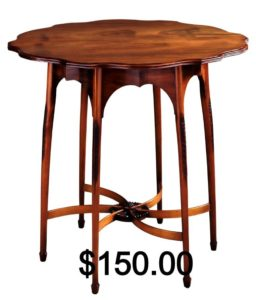## Putting It Together: Polynomial and Rational Expressions

At the beginning of the module, we discussed a hypothetical furniture company that manufactures and sells a certain kind of chair at $40. Let’s recall the revenue (R), cost (C) and profit (P) formulas in terms of the number of chairs sold ($x$). We will write each one in standard form. $R=40x$ $C=0.001x^2+25x+10,000$ $P=-0.001x^2+15x-10,000$ Each of these three formulas is a polynomial. The revenue is degree 1, while the cost and profit are both degree 2.Now suppose our company also makes tables to go with the chairs priced at$150. The revenue for making and selling $x$ tables would then be $R=150x$. Materials for the table cost $80 per unit. If our factory is already making chairs, then there is no need to count the fixed costs again in table production costs, so our total cost formula for $x$ tables is: $C=0.002x^2+80x$ The degree 2 term comes from some additional analysis of the costs. Don’t worry about where this term comes from; instead, think of it as a given quantity. For simplicity, we’re going to assume that tables and chairs are sold as a set (one table and one chair per set). What is the total profit generated when 100 sets are built and sold? What about 200? Because each set consists of one table and one chair, the total revenue generated by selling $x$ sets can be found by adding the revenue polynomials. $R=40x+150x=190x$ Furthermore, the total costs of manufacturing $x$ sets is found by adding the cost polynomials. $C=\left(0.001x^2+25x+10,000\right)+\left(0.002x^2+80x\right)$ $C=0.003x^2+105x+10,000$ Finally, we find the profit by subtracting polynomials, $P=R-C$. Don’t forget to distribute the minus sign and then collect like terms. $P=190x-\left(0.003x^2+105x+10,000\right)$ $P=190x-0.003x^2-105x-10,000$ $P=-0.003x^2+85x-10,000$ So how much profit results from making and selling 100 sets? Plug in $x=100$ to find out. $P=-0.003\left(100\right)^2+85\left(100\right)-10,000=-1530$ That negative value means that we lost$1,530 on this project!  But if you think about it, this result probably means we just haven’t made enough of our product to cover the fixed costs. Let’s see if increasing the production helps our bottom line. What is the profit in making and selling 200 table-chair sets?

$P=-0.003\left(200\right)^2+85\left(200\right)-10,000=6880$

This time our company has made $6,880 in profit. Not bad for one month of work. But let’s dig a little deeper. What is the average profit per set? First set up the formula (which is an example of a rational function). $\Large\frac{P}{x}=\frac{-0.003x^2+85x-10,000}{x}$ For example, the average profit when $x=200$ is: $\Large\frac{-0.003\left(200\right)^2+85\left(200\right)-10,000}{200}=\frac{6880}{200}=34.4$ That means that we are making roughly$34.40 in profit per set.  Below you can see how the average profit changes when more sets are produced and sold. About how many sets would we have to make in order to get the most average profit?

 Number of sets $x$ Average profit per set $\large\frac{P}{x}$ 200 $34.40 400$58.80 600 $66.53 800$70.10 1000 $72.00 1200$73.07 1400 $73.66 1600$73.95 1800 $74.04 2000$74.00 2200 $73.85 Table shows the correlation between the number of sets sold and the profit per set. According to our table, the highest average profit of$74.04 occurs when roughly 1800 sets are produced and sold. Looks like we need to increase production!